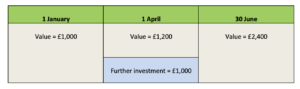JUNE 2021
EDITION

Register with PP

Newsletter, Jobs & Event Alerts

## Latest

Exam refresher: Money-weighted & time-weighted rate of return

23 June 2020Catriona Standingford, MD of Brand Financial Training, considers how to calculate money-weighted rate of return and time-weighted rate of return when evaluating performance of an investment portfolio. This calculation can arise in a number of CII exams, notably R02 and AF4.

Anyone sitting one of the CII investment exams will need to be familiar with measuring returns.

In this article we look at the money-weighted rate of return (MWR) and the time-weighted rate of return (TWR) both of which are examined in R02 as well as in J10, J12 and AF4; in particular in AF4 students may need to actually do the calculations whereas in R02 it will be more about understanding the theory.

• The time-weighted return shows the performance regardless of the timing of new monies.
• The money-weighted return takes account of timing of additions and withdrawals.

Let’s look at a typical exam question:

Calculate the money-weighted rate of return for the following investment:Where:

• D = income received during the year i.e. £2,000
• = the value at the end of the period i.e. £119,000
• = the value at the start of the period i.e. £100,000
• C = the new money introduced during the year i.e. £20,000
• n = the number of months remaining in the year i.e. 31 March until the 31 December

If we input the figures into the formula:

£2,000 + £119,000 – £100,000 – £20,000 /£100,000 + (£20,000 x 9/12)

Therefore:

£1,000 / £115,000 x 100 = MWR of 0.87 or 0.87%

The MWR is influenced by the timing of cash flows which are of course outside the fund manager’s control.

The TWR aims to eliminate this problem by measuring compound growth irrespective of money flows.  To calculate TWR we need to know when and how much new money is invested, when and how much of a withdrawal is made and what the portfolio value was at the time.  Returns are then calculated for each period between these contributions or withdrawals and then the periods are all multiplied together.

Let’s look at an example of this:What is the TWR?

Period 1 = This is the first three months when the fund went from £1,000 to £1,200.

Period 2 = This is the next three months when £1,200 plus the additional investment of £1,000 grew to £2,400.

The return for each period is calculated by dividing the end value by the value at the beginning.

Period 1 = £1,200/£1,000 = 1.2

Period 2 = £2,400/£2,200 = 1.0909

So now we know that in the first 3 months a gain of 20% was made and in the second 3 months a gain of 9.09% was made.  To find the TWR we need to multiply the two periods together:

(1.2) x (1.0909) – 1 x 100 = 30.91%

In conclusion, both the MWR and the TWR are useful when evaluating performance of an investment portfolio over a given time period.  However, TWR focuses just on performance and is therefore more suitable when comparing different portfolio returns.

First published in the March 2020 issue of Professional Paraplanner